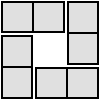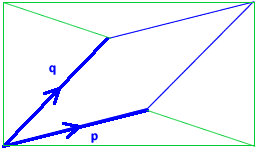#### You may also like### Teddy Town

There are nine teddies in Teddy Town - three red, three blue and three yellow. There are also nine houses, three of each colour. Can you put them on the map of Teddy Town according to the rules?### 4 Dom

Use these four dominoes to make a square that has the same number of dots on each side.### Just Rolling Round

P is a point on the circumference of a circle radius r which rolls, without slipping, inside a circle of radius 2r. What is the locus of P?

# Areas from Vectors

##### Age 11 to 16

Tony computed the answers for some particular cases.

For $\mathbf{p}=\left(\begin{array}{c}3\\ 0\end{array}\right), \mathbf{q}=\left(\begin{array}{c}5 \\ 2\end{array}\right)$ the area is $6$ units squared ($=3\times 2-0\times 5$)
For $\mathbf{p}=\left(\begin{array}{c}3 \\ 2\end{array}\right), \mathbf{q}=\left(\begin{array}{c}0 \\ 4\end{array}\right)$ the area is $12$ units squared ($=3\times 4-2\times 0$)
For $\mathbf{p}=\left(\begin{array}{c}4 \\ 1\end{array}\right), \mathbf{q}=\left(\begin{array}{c}3 \\ 3\end{array}\right)$ the area is $9$ units squared ($=4\times 3-1\times 3$)
For $\mathbf{p}=\left(\begin{array}{c}2 \\ 4\end{array}\right), \mathbf{q}=\left(\begin{array}{c}-1 \\ 3\end{array}\right)$ the area is $10$ units squared ($=2\times 3-4\times (-1)$)

James, Jamie and George from Gillingham School sent us their formula for the area of the general parallelogram.

$$a d-b c\ \text{ (or } bc-ad\text{ if } bc>ad)$$

Here's one way to work that out.Remember that $\mathbf{p}=\left(\begin{array}{c}a \\ b\end{array}\right)$ and $\mathbf{q}=\left(\begin{array}{c}c\\d\end{array}\right)$.

The diagram shows the parallelogram inside a rectangle with base $a+c$ and height $b+d$. This rectangle has area $(a+c)(b+d)$. If we could find the areas of the triangles outside the parallelogram, then we could subtract them from the area of the rectangle to find the area of the parallelogram.

The bottom triangle has height $b$ and base $a+c$, so has area $\frac{1}{2}b(a+c)$.

The triangle on the left has (if you imagine it on its side so that its base is at the bottom) base $b+d$ and height $c$, so has area $\frac{1}{2}c(b+d)$.

The triangle at the top is congruent to the triangle at the bottom (it has been rotated by $180^{\circ}$), so has area $\frac{1}{2}b(a+c)$.

The triangle on the right is congruent to the triangle on the left, so has area $\frac{1}{2}c(b+d)$.

So the area of the parallelogram is $$(a+c)(b+d)-2\times\frac{1}{2}b(a+c) -2\times\frac{1}{2}c(b+d)=$$
$$a b+b c+a d+c d-a b-b c-b c-c d=a d-b c$$

just as James, Jamie and George said.

Yingtong Yan solved the problem differently, using  stage 5 mathematics.

If we let $\mathbf{p}(x_1, y_1)$ and $\mathbf{q}(x_2, y_2)$ be our two vectors, then the cosinus of the angle between them is
$$\cos<\mathbf{p},\mathbf{q}>=\frac{\mathbf{p} \cdot \mathbf{q}}{|\mathbf{p}|\times |\mathbf{q}|}.$$
Therefore, $$\cos^2<\mathbf{p},\mathbf{q}>=\frac{(\mathbf{p} \cdot \mathbf{q})^2}{(|\mathbf{p}|\times |\mathbf{q}|)^2}$$
and, since $\sin^2<\mathbf{p},\mathbf{q}>+\cos^2<\mathbf{p},\mathbf{q}>=1$,
$$\sin^2<\mathbf{p},\mathbf{q}>=1 - \frac{(\mathbf{p} \cdot \mathbf{q})^2}{(|\mathbf{p}|\times |\mathbf{q}|)^2}= \frac{(|\mathbf{p}|\times |\mathbf{q}|)^2-(\mathbf{p} \cdot \mathbf{q})^2}{(|\mathbf{p}|\times |\mathbf{q}|)^2}.$$

Moreover, since the angle between $\mathbf{p}$ and $\mathbf{q}$ is smaller then $180^\circ$, then $\sin<\mathbf{p},\mathbf{q}>$ is greater then 0, and, hence, $\sin<\mathbf{p},\mathbf{q}> = \sqrt{\frac{(|\mathbf{p}|\times |\mathbf{q}|)^2-(\mathbf{p}\cdot\mathbf{q})^2}{(|\mathbf{p}|\times |\mathbf{q}|)^2} }$.

Using the fact that the parallelogram is made out of two congruent triangles of area $\frac{1}{2}\times\sin<\mathbf{p},\mathbf{q}>\times |\mathbf{p}|\times |\mathbf{q}|$, the area of the parallelogram is
\begin{aligned} A_{\text{parallelogram}} &= 2\times \frac{1}{2}\times\sin<\mathbf{p},\mathbf{q}>\times |\mathbf{p}|\times |\mathbf{q}| \\ &= \sqrt{\frac{(|\mathbf{p}|\times |\mathbf{q}|)^2-(\mathbf{p}\cdot\mathbf{q})^2}{(|\mathbf{p}|\times |\mathbf{q}|)^2} }\times |\mathbf{p|}\times |\mathbf{q}| \\ &= \sqrt{ (|\mathbf{p}|\times |\mathbf{q}|)^2-(\mathbf{p}\cdot\mathbf{q})^2} \\ &= \sqrt{(x_1^2+y_1^2)\times (x_2^2+y_2^2) - (x_1x_2+y_1y_2)^2} \\ &=\sqrt{x_1^2y_2^2+x_2^2y_1^2-2x_1x_2y_1y_2} \\ &=|x_1y_2-x_2y_1| \end{aligned}

Beth and Susie analyzed the special case when the parallelogram is a square.

If the parallelogram is a square then $|\mathbf{p}|=|\mathbf{q}|$ and the area is $|\mathbf{p}|^2$. Since $|\mathbf{p}|=\sqrt{a^2+b^2}$, the area is $\sqrt{a^2+b^2}^2=a^2+b^2$.

Well done to everyone who sent in a solution. Keep exploring!